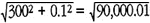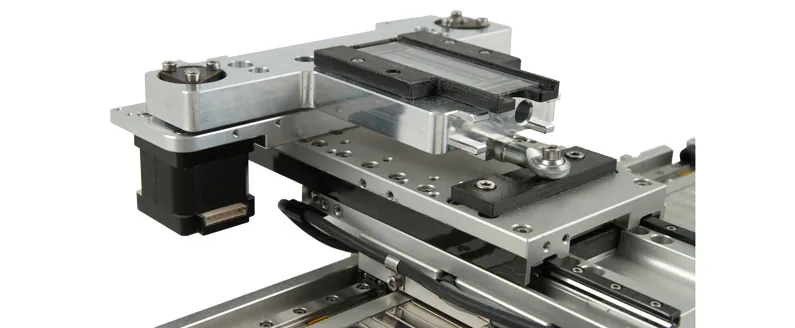# Cosine Error

## Cosine error results from an angular misalignment between the motion of a positioning table, and the accuracy determining element (leadscrew, encoder, or laser interferometer beam path).

Under most circumstances, it has a negligible effect on overall accuracy, owing to the significant degree of misalignment needed to influence accuracy. Consider, for example, the case of a 300 mm travel positioning table with a linear encoder. The encoder is pitched so as to be inclined to the direction of motion, and the encoder will accordingly measure a larger move than has actually occurred. Pythagoras’s theorem (a2 + b2 = c2) yields the magnitude of the error. At a 100 micron misalignment, the encoder path equals,or 300.0000167; the error is only 17 nanometers. If the misalignment is specified in terms of angle, then the error will equal: travel x (1 – cosine of theta), hence the name, cosine error. In the above example, the angle was 68.75 arc-seconds, and the cosine of theta equals 0.999999944.

If the encoder resolution is one micron, then a misalignment of 800µm would be necessary to generate a cosine error equivalent to a single count. Our stage design, fixturing, and inspection procedures hold leadscrew and encoder alignment to levels far below this value, rendering cosine error of negligible consequence in most positioning stages. In systems using laser interferometers for positional feedback however, simple visual alignment with a reduced aperture can introduce cosine error on the order of 5 ppm. This is significant when compared with the intrinsic interferometer accuracy of <1 ppm, and may necessitate careful adjustment of the beam angle in pitch and yaw to maximize the measured distance. Note that with laser interferometers, cosine error results in a distance measurement smaller than the actual move; this is opposite to the effect of cosine error for a linear encoder.The longer the travel of the positioning stage, the easier it is to control cosine error.  One case in which cosine error can be difficult to minimize is in cases where the stage travel is very small.  For example, in a flexure stage with a total travel of 100 um, it can be difficult to precisely align the motion axis and that of the accuracy determining element. In these cases, optical alignment techniques can be used to minimize the effect of cosine error